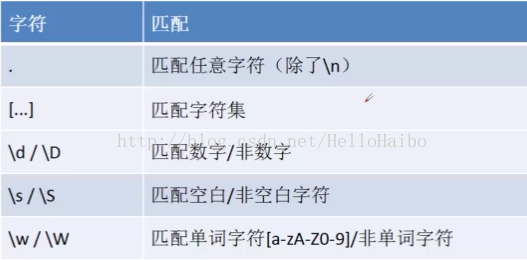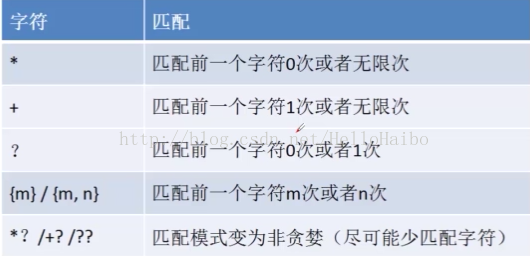### 1、纯字符串匹配：

 1234 import re str = "hello,everyone,I am HelloHaibo,thank u for watching my blog" pattern = re.compile(r'Haibo') content = re.findall(pattern,str) 
 1 content: ['Haibo'] 

### 2、单字符匹配：

 1234 import re str = "hello,everyone,I am HelloHaibo,thank u for watching my blog" pattern = re.compile(r'.ello') content = re.findall(pattern,str) 
1
 1 content: ['hello', 'Hello'] 

 1234 import re str2 = "1 + 2 = 3,yeah" pattern = re.compile(r'\d') content = re.findall(pattern,str2) 
1
 1 content: ['1', '2', '3'] 

 1234 import re str2 = "1 + 2 = 3,yeah" pattern = re.compile(r'\D') content = re.findall(pattern,str2) 
 1 content: [' ', '+', ' ', ' ', '=', ' ', ',', 'y', 'e', 'a', 'h'] 

 1234 import re str2 = "1 + 2 = 3,Yeah" pattern = re.compile(r'\w') content = re.findall(pattern,str2) 
 1 content: ['1', '2', '3', 'Y', 'e', 'a', 'h'] 

\w把字符串里的数字，小写字母，大写字母都匹配出来了。

 1234 import re str2 = "1 + 2 = 3,Yeah" pattern = re.compile(r'\W') content = re.findall(pattern,str2) 
 1 content: [' ', '+', ' ', ' ', '=', ' ', ','] 

\W把除了数字，小写字母，大写字母以外的都匹配出来了。### 3、单字符匹配：

 1234 import re str2 = "1 + 2 = 3,yeah" pattern = re.compile(r'[0-4]') content = re.findall(pattern,str2) 
1
 1 content: ['1', '2', '3'] 
 1234 import re str2 = "1 + 2 = 3,yeah" pattern = re.compile(r'[a-z]') content = re.findall(pattern,str2) 
 1 content: ['y', 'e', 'a', 'h'] 

• * 匹配前一个字符零次或者无限次
 1234 import re str1 = "http https httpes httpsss" pattern = re.compile(r'https*') content = re.findall(pattern,str1) 
 1 content: ['http', 'https', 'http', 'httpsss'] 
• + 表示匹配前面的字符一次或者无限次
 1234 import re str1 = "http https httpes httpsss" pattern = re.compile(r'https+') content = re.findall(pattern,str1) 
 1 content: ['https', 'httpsss'] 

• ? 表示匹配前一个字符零次或者一次
 1234 import re str1 = "http https httpsss" pattern = re.compile(r'https?') content = re.findall(pattern,str1) 
 1 content: ['http', 'https', 'https'] 

 1234 import re str1 = "http https httpsss" pattern = re.compile(r'https{0,1}') content = re.findall(pattern,str1) 
 1 content: ['http', 'https']1234 import re str1 = "hello,everyone,I am HelloHaibo,thank u for watching my blog" pattern = re.compile(r'[Hh]ello') content = re.findall(pattern,str1) 
1
 1 content: ['hello', 'Hello'] 

 1234 import re str1 = "

www.baidu.com

www.imooc.com

tttt" pattern = re.compile(r'www\.[a-zA-Z]+\.com') content = re.findall(pattern,str1) 
 1 content: ['

www.baidu.com

', '

www.imooc.com

'] 

### 4、断言匹配(这个里面的核心思想是判断):

(?<=...)(?=...)两个分别称为后向匹配和前向匹配，它们判断是否成立，但是不参与匹配输出。

 1234 import re str1 = "

www.baidu.com

www.imooc.com

tttt" pattern = re.compile(r'(?<=)www\.[\w]+\.com(?=)') content = re.findall(pattern,str1) 
 1 content: ['www.baidu.com', 'www.imooc.com'] 

《正则表达式必知必会（修正版）》